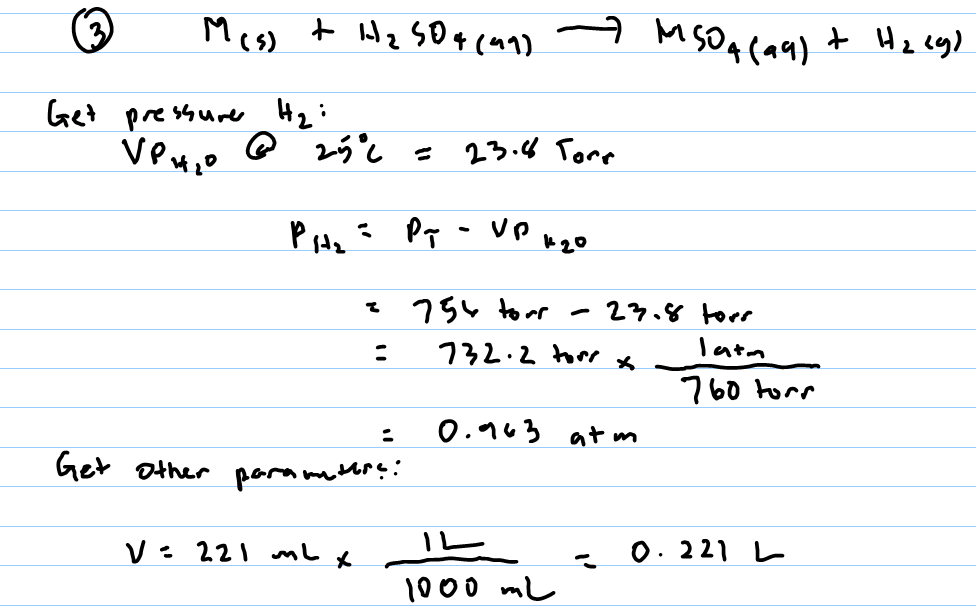# Problem: A 0.486 gram sample of a metal, M, reacts completely with sulfuric acid according to:M(s) + H2SO4(aq) → MSO4(aq) + H2(g)A volume of 221 mL of hydrogen is collected over water; the water level in the collecting vessel is the same as the outside level. Atmospheric pressure is 756.0 Torr and the temperature is 25°C. The vapor pressure of water at various temperatures can be found in this table. Calculate the molar mass of the metal.

###### FREE Expert Solution###### Problem Details

A 0.486 gram sample of a metal, M, reacts completely with sulfuric acid according to:

M(s) + H2SO4(aq) → MSO4(aq) + H2(g)

A volume of 221 mL of hydrogen is collected over water; the water level in the collecting vessel is the same as the outside level. Atmospheric pressure is 756.0 Torr and the temperature is 25°C. The vapor pressure of water at various temperatures can be found in this table. Calculate the molar mass of the metal.

What scientific concept do you need to know in order to solve this problem?

Our tutors have indicated that to solve this problem you will need to apply the Collecting Gas Over Water concept. If you need more Collecting Gas Over Water practice, you can also practice Collecting Gas Over Water practice problems.

What is the difficulty of this problem?

Our tutors rated the difficulty ofA 0.486 gram sample of a metal, M, reacts completely with su...as high difficulty.

How long does this problem take to solve?

Our expert Chemistry tutor, Dasha took 8 minutes and 29 seconds to solve this problem. You can follow their steps in the video explanation above.

What professor is this problem relevant for?

Based on our data, we think this problem is relevant for Professor Mandelshtam's class at UCI.